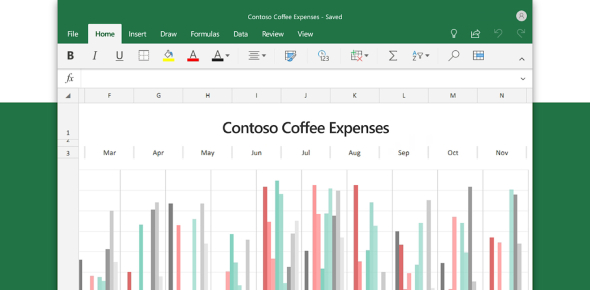17 Questions | Attempts: 4596
ShareSettings• 1.
The place in a spreadsheet where a column and row intersect
• A.

Row

• B.

Column

• C.

Cell

• D.

Entry bar

• 2.
The part of the spreadsheet that is always called by the letter
• A.

Row

• B.

Column

• C.

Cell

• D.

Entery bar

• 3.
The part of the spreadsheet that is always called by a number
• A.

Row

• B.

Column

• C.

Cell

• D.

Entery bar

• 4.
Place on the spreadsheet were information is typed
• A.

Pointer

• B.

• C.

Formulas

• D.

Data entery bar

• 5.
Place on the spreadsheet that tells the address of the active cell
• A.

Column

• B.

Row

• C.

• D.

Data entery bar

• 6.
This mouse pointer is used to
• A.

Copy or create a series of numbers, dates, days

• B.

Select a range of cells

• C.

Move the contents of a cell or a range of cells

• D.

Resize columns and rows

• E.

Select a whole coloumn or row

• 7.
This mouse pointer is used to
• A.

Copy or create a series of numbers, dates, days

• B.

Select a range of cells

• C.

Move the contents of a cell or a range of cells

• D.

Resize columns and rows

• E.

Select a whole coloumn or row

• 8.
This mouse pointer is used to
• A.

Copy or create a series of numbers, dates, days

• B.

Select a range of cells

• C.

Move the contents of a cell or a range of cells

• D.

Resize columns and rows

• E.

Select a whole coloumn or row

• 9.
This mouse pointer is used to
• A.

Copy or create a series of numbers, dates, days

• B.

Select a range of cells

• C.

Move the contents of a cell or a range of cells

• D.

Resize columns and rows

• E.

Select a whole coloumn or row

• 10.
This mouse pointer is used to
• A.

Copy or create a series of numbers, dates, days

• B.

Select a range of cells

• C.

Move the contents of a cell or a range of cells

• D.

Resize columns and rows

• E.

Select a whole coloumn or row

• 11.
A grid of rows and columns is called a ________.
• 12.
Used to display information and solve problems involving numbers
• 13.
What symbol in the data entry bar tells the spread sheet you are entering a formula?
• A.

+

• B.

-

• C.

*

• D.

/

• E.

=

• 14.
You can use the following keys to move from cell to cell : arrow keys, tab, enter.
• A.

True

• B.

False

• 15.
Three types of data found in a cell are numbers, formulas, and equations.
• A.

True

• B.

False

• 16.
One spreadsheet is sometimes called a
• A.

Formula

• B.

Column

• C.

Worksheet

• D.

Row

• 17.
One or more worksheets together in a file is called aBack to top